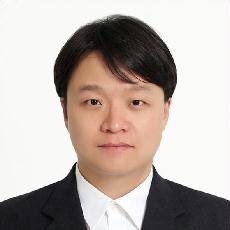###정보이름최수영 학력박사 전공수학전공(과) 연구실 : 팔달관 601 연구실번호 : 3322 이메일 : schoi@ajou.ac.kr 연구관심분야 : Toric topology 홈페이지 : http://ajou.ac.kr/~schoi 졸업연도/학교/학위 2009.01 한국과학기술원 박사 졸업연도/학교/학위 2003.02 한국과학기술원 학사 2015년 1월 1일 - 현재 :  대한수학회 사업이사2015년 3월 1일 - 현재 :  대한수학회 위상수학 분과위원 2013년 상산 젊은 수학자상 (대한수학회)2012년 청암 과학 펠로 (포스코 청암재단)2009년 JSPS Postdoctoral Research Fellowship (일본학술진흥회) 연구활동(주요논문) [논문] Strong cohomological rigidity of toric varieties (PROCEEDINGS OF THE ROYAL SOCIETY OF EDINBURGH SECTION A-MATHEMATICS) - 2017.10 최수영, 박선정 [논문] On the cohomology and their torsion of real toric objects (FORUM MATHEMATICUM) - 2017.05 최수영, 박한철 [논문] Classification of real Bott manifolds and acyclic digraphs (TRANSACTIONS OF THE AMERICAN MATHEMATICAL SOCIETY) - 2017.04 최수영, 엄상일, MIkiya Masuda [논문] Classification of Bott manifolds up to dimension 8 (PROCEEDINGS OF THE EDINBURGH MATHEMATICAL SOCIETY) - 2015.10 최수영 [논문] Combinatorial Rigidity of 3-dimensional Simplicial Polytopes (INTERNATIONAL MATHEMATICS RESEARCH NOTICES) - 2011.04 최수영, 김장수 국제학술논문지 [논문] The Betti Numbers of Real Toric Varieties Associated to Weyl Chambers of Type B (CHINESE ANNALS OF MATHEMATICS SERIES B) - 2017.11 최수영, 박한철, 박보람 [논문] Strong cohomological rigidity of toric varieties (PROCEEDINGS OF THE ROYAL SOCIETY OF EDINBURGH SECTION A-MATHEMATICS) - 2017.10 최수영, 박선정 [논문] Wedge operations and torus symmetries II (CANADIAN JOURNAL OF MATHEMATICS-JOURNAL CANADIEN DE MATHEMATIQUES) - 2017.08 최수영, 박한철 [논문] On the cohomology and their torsion of real toric objects (FORUM MATHEMATICUM) - 2017.05 최수영, 박한철 [논문] Wedge operations and a new family of projective toric manifolds (ISRAEL JOURNAL OF MATHEMATICS) - 2017.04 최수영, 박한철 [논문] Pseudograph and its associated real toric manifold (JOURNAL OF THE MATHEMATICAL SOCIETY OF JAPAN) - 2017.04 최수영, 박선정, 박보람 [논문] Classification of real Bott manifolds and acyclic digraphs (TRANSACTIONS OF THE AMERICAN MATHEMATICAL SOCIETY) - 2017.04 최수영, 엄상일, MIkiya Masuda [논문] Homotopy decomposition of a suspended real toric space (Boletin de la Sociedad Matematica Mexicana) - 2016.03 최수영, Stephen Theriault, Shizuo Kaji [논문] Wedge operations and torus symmetries (TOHOKU MATHEMATICAL JOURNAL) - 2016.03 최수영, 박한철 [논문] Projective bundles over toric surfaces (INTERNATIONAL JOURNAL OF MATHEMATICS) - 2016.03 최수영, 박선정 [논문] Classification of Bott manifolds up to dimension 8 (PROCEEDINGS OF THE EDINBURGH MATHEMATICAL SOCIETY) - 2015.10 최수영 [논문] Invariance of Pontrjagin classes for Bott manifolds (ALGEBRAIC AND GEOMETRIC TOPOLOGY) - 2015.04 최수영, Satsohi Murai, Mikiya Masuda [논문] A new graph invariant arises in toric topology (JOURNAL OF THE MATHEMATICAL SOCIETY OF JAPAN) - 2015.04 최수영, 박한철 [논문] Introduction to toric topology (ASIA PACIFIC MATHEMATICS NEWSLETTER) - 2014.07 최수영 [논문] Different moment-angle manifolds arising from two polytopes having the same bigraded Betti numbers (ALGEBRAIC AND GEOMETRIC TOPOLOGY) - 2013.10 최수영 [논문] Classification of Q-trivial Bott manifolds (JOURNAL OF SYMPLECTIC GEOMETRY) - 2012.09 최수영, Mikiya Masuda [논문] Strong cohomological rigidity of a product of projective spaces (BULLETIN OF THE KOREAN MATHEMATICAL SOCIETY) - 2012.07 최수영, 서동엽 [논문] TOPOLOGICAL CLASSIFICATION OF QUASITORIC MANIFOLDS WITH SECOND BETTI NUMBER 2 (PACIFIC JOURNAL OF MATHEMATICS) - 2012.05 최수영, 서동엽, 박선정 [논문] Rigidity Problems in Toric Topology: A Survey (PROCEEDINGS OF THE STEKLOV INSTITUTE OF MATHEMATICS) - 2011.12 최수영, Mikiya Masuda, 서동엽 [논문] Topological classification of torus manifolds which have codimension one extended actions (ALGEBRAIC AND GEOMETRIC TOPOLOGY) - 2011.09 최수영, Shintaro Kuroki [논문] Combinatorial Rigidity of 3-dimensional Simplicial Polytopes (INTERNATIONAL MATHEMATICS RESEARCH NOTICES) - 2011.04 최수영, 김장수 [논문] Properties of Bott manifolds and cohomological rigidity (ALGEBRAIC AND GEOMETRIC TOPOLOGY) - 2011.03 최수영, 서동엽 [논문] Toric cohomological rigidity of simple convex polytopes (Journal of London Mathematical Society) - 2010.10 최수영, Taras Panov, 서동엽 [논문] The number of orientable small covers over cubes (PROCEEDINGS OF THE JAPAN ACADEMY SERIES A-MATHEMATICAL SCIENCES) - 2010.06 최수영 [논문] Quasitoric manifolds over a product of simplices (Osaka Journal of Mathematics) - 2010.03 최수영, 서동엽, Mikiya Masuda [논문] Topological classification of generalized Bott towers (TRANSACTIONS OF THE AMERICAN MATHEMATICAL SOCIETY) - 2010.02 최수영, Mikiya Masuda, 서동엽 [논문] A combinatorial proof of a formula for Betti numbers of a stacked polytope (The electronic journal of combinatorics) - 2010.01 최수영, 김장수 국내학술논문지 [논문] 초등학생의 수학학습 포기 인식과 정의적 요인 연관성 분석 (초등수학교육) - 2017.05 고호경, 김형원, Kaji, Shizuo, 최수영